Constructing a triangle when 3 sides known (SSS)

Practical Geometry
Concept wise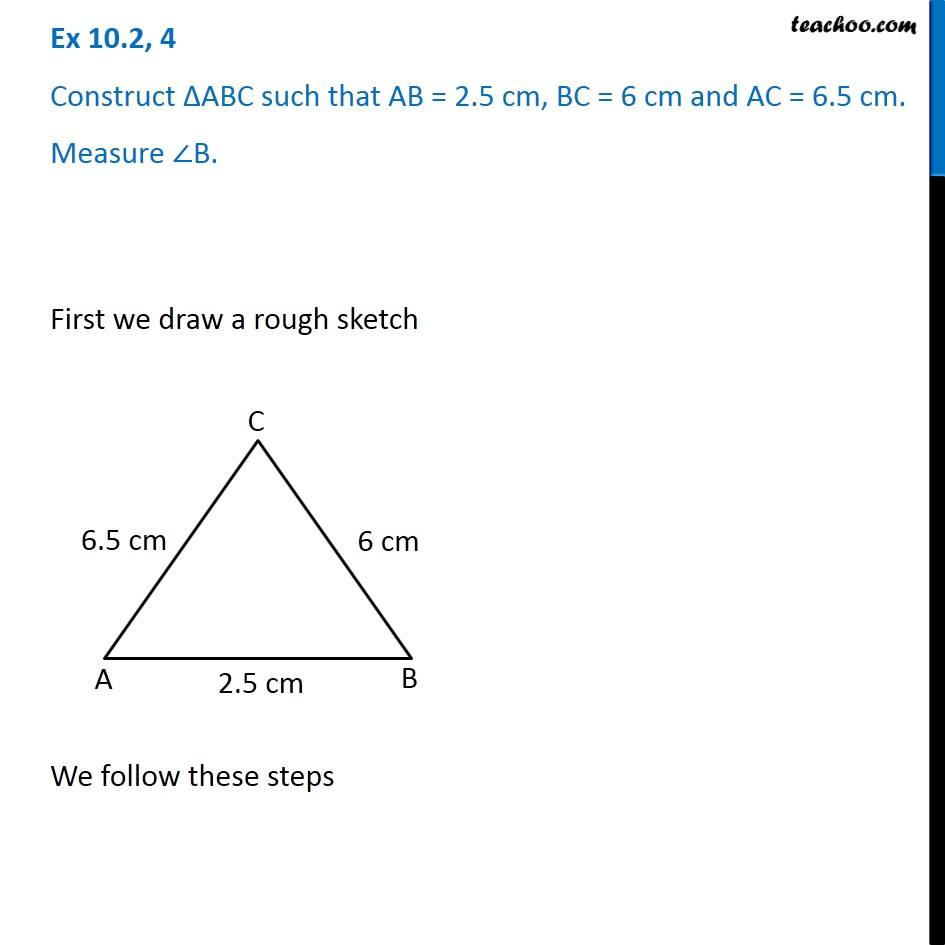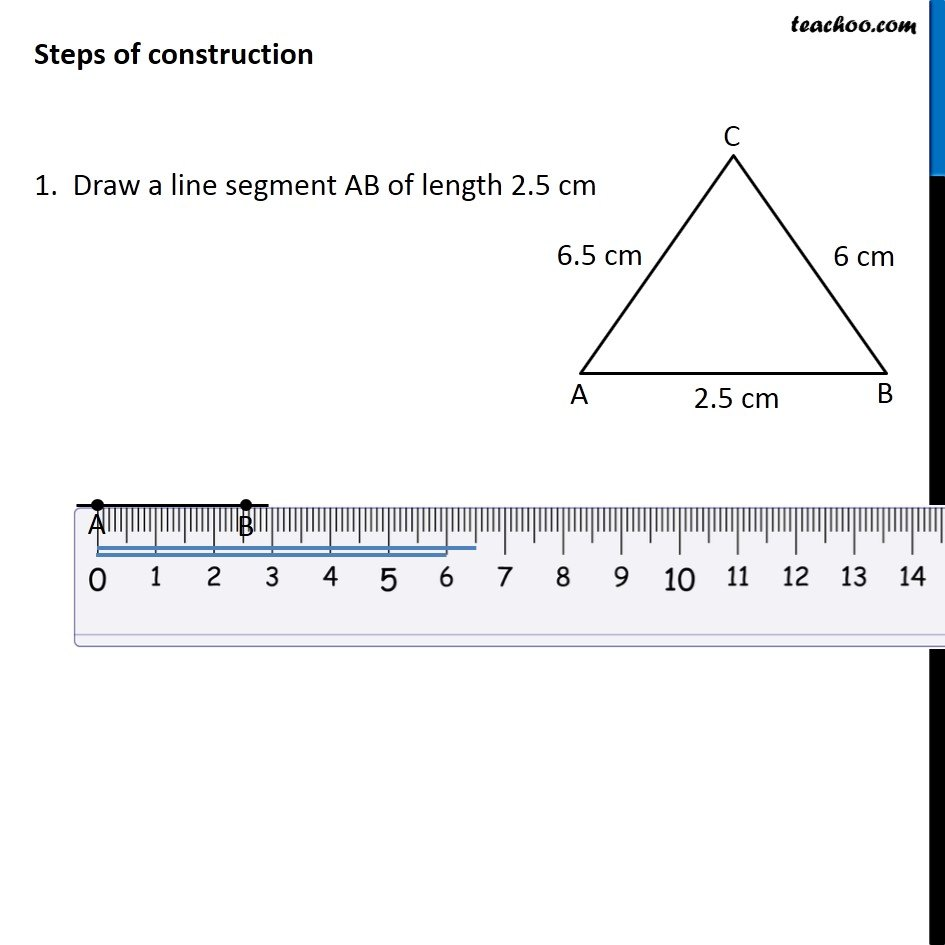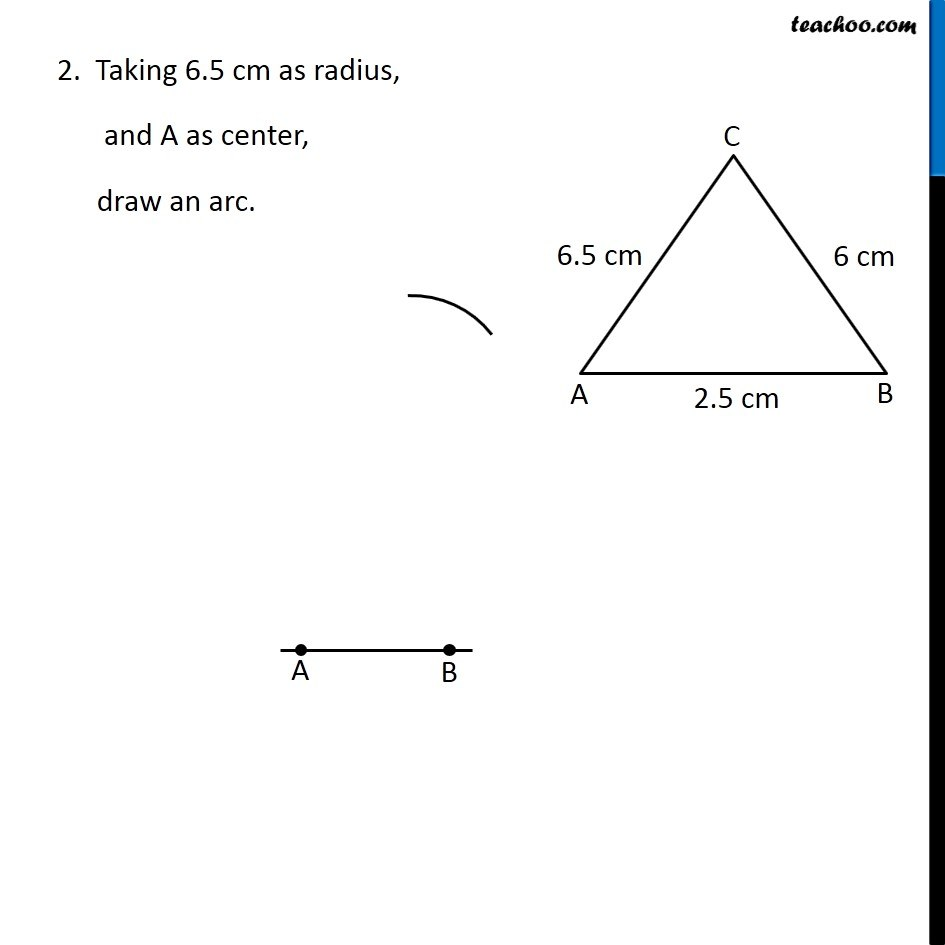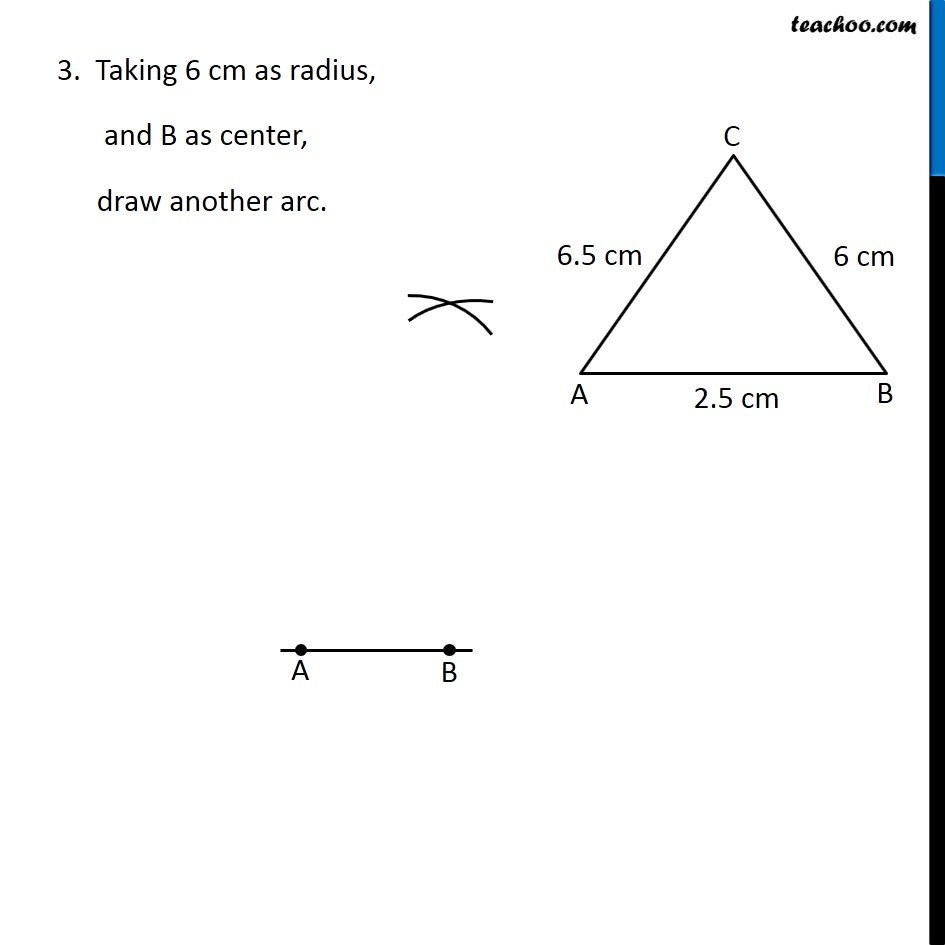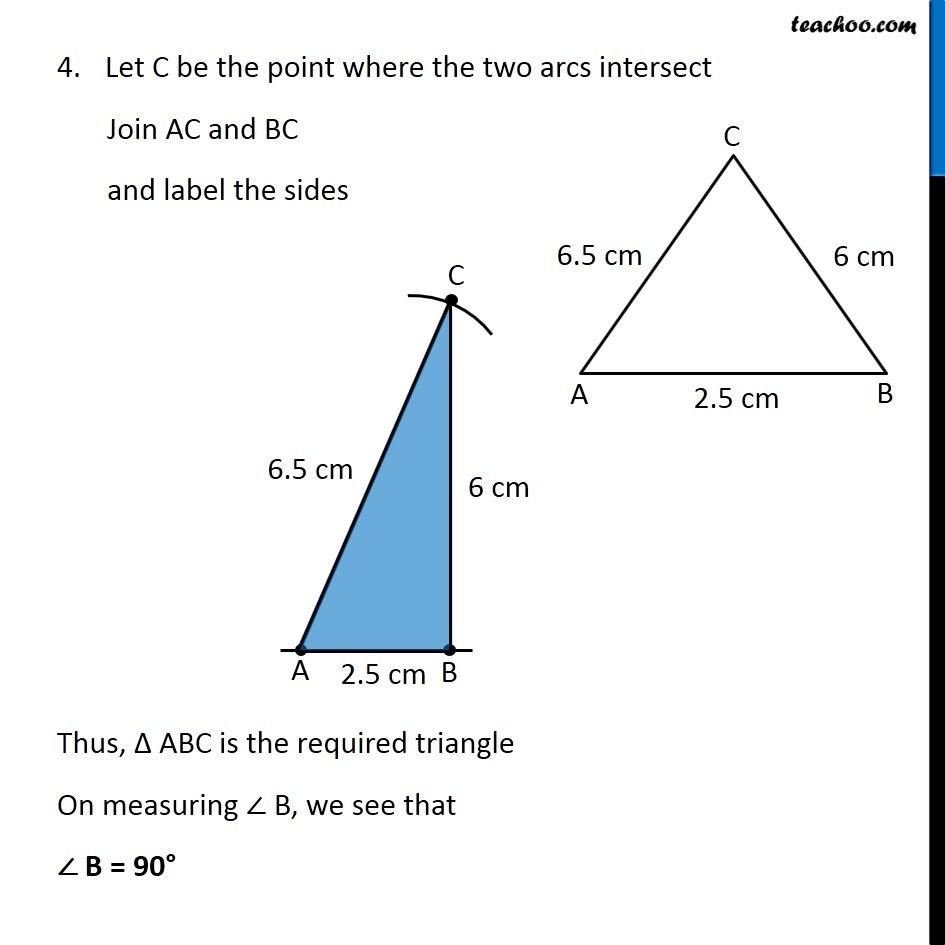Learn in your speed, with individual attention - Teachoo Maths 1-on-1 Class

### Transcript

Question 4 Construct ∆ABC such that AB = 2.5 cm, BC = 6 cm and AC = 6.5 cm. Measure ∠B. First we draw a rough sketch We follow these steps Steps of construction 1. Draw a line segment AB of length 2.5 cm 2. Taking 6.5 cm as radius, and A as center, draw an arc. 3. Taking 6 cm as radius, and B as center, draw another arc. 4. Let C be the point where the two arcs intersect Join AC and BC and label the sides Thus, Δ ABC is the required triangle On measuring ∠ B, we see that ∠ B = 90°